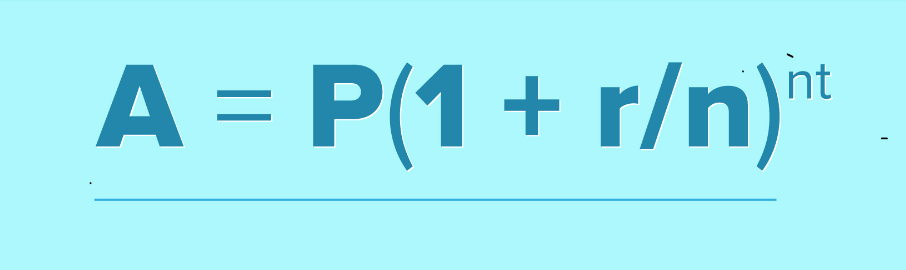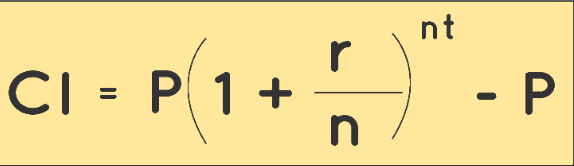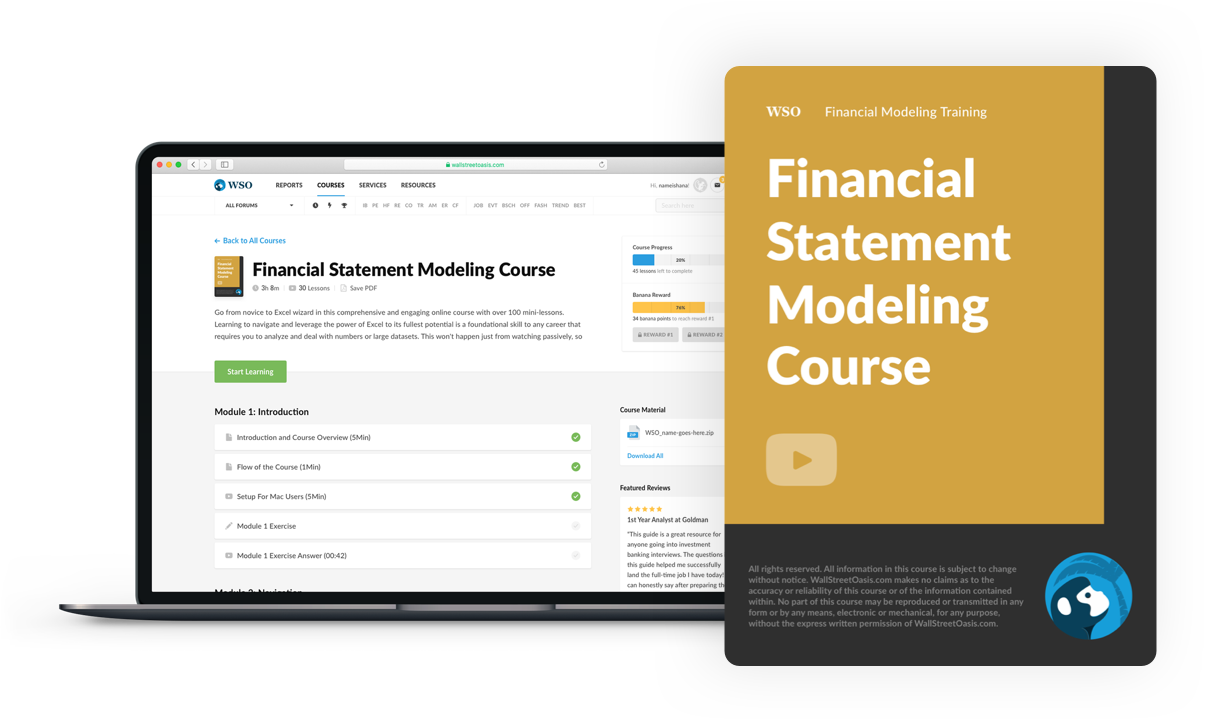# Compound Interest Formula

It is the interest on a loan or deposit that is calculated using both the initial principle

The interest on a loan or deposit that is calculated using both the initial principle and the accrued interest from prior periods is known as compound interest (or compounding interest). Compound interest often referred to as "interest on interest," is said to have started in 17th-century Italy.It will cause a payment to grow more quickly than simple interest, which is computed just on the principal amount. The frequency of compounding determines the pace at which compound interest accumulates, the more compounding periods, the higher the compound interest.

The yearly interest rate is raised to the number of compound periods minus one, and the starting principal amount is multiplied by both of these factors. The resulting value is subsequently deducted from the loan's entire original amount.

If we examine our bank statements, we will typically see that our account is credited with interest on a yearly basis. Even while the principal remains the same, the interest changes annually. We can observe that interest rises over time.

As a result, we can infer that the interest the bank charges is compound interest, or CI, rather than simple interest. You will discover what CI is in this article, along with the definition and derivation of the formula used to determine CI, whether compounded annually, biannually, quarterly, etc.

The majority of transactions in the banking and financial industries, as well as other areas, use compound interest. Some of its uses include:

• Population growth or decline.

• The expansion of bacteria.

• Increase or decrease in an item's value.

Similar to compound interest, simple interest is a quick and simple formula for figuring out how much interest will be charged on loan. Simple interest is calculated by multiplying the daily interest rate, the principle, and the number of days between payments.

## How to calculate compound interest?

Compound interest can be calculated in math in a variety of ways, depending on the circumstances. To make the calculations simpler, we can utilize the compound interest formula.

We need to know the quantity and the principal in order to compute compound interest. The difference between the two is the principal.

Compound interest, as we've already discussed, is interest calculated using both the initial principal and interest accrued over time. The following is the principal compound interest formula:

Amount - Principal = Compound Interest

Principal is the whole borrowed (or invested) sum, exclusive of interest and income. The entire amount repaid at the conclusion of the borrowing period is indicated by the letter A.

The formula for the amount is as follows:Where,

A = Amount

P = principal amount

r = annual interest rate, which is described in decimal numbers

n = it is the number of times the interest is compounded per time period

t = the amount of time the money is saved or borrowed

For example, if \$5,000 is deposited into a savings account with a monthly compound interest rate of 5%, the investment's worth after 10 years can be calculated as follows:

Step 1

Writing the values given in the question -

P = 5000

r = 5/100 = 0.05 (decimal)

n = 12

t = 10

Step 2

These are the results we receive when we enter those numbers into the formula of amount -

A= P(1+r/n)^nt

A = 5000 (1 + 0.05 / 12) (12 * 10) = 8235.05.

The investment balance is, therefore, \$8,235.05

Step 3

To now find the compound interest value will be:

CI = A - P

CI = 8,235 - 5000

CI = \$ 3,235

Therefore, we can conclude that the formula for compound interest is:Over the long term, compound interest can greatly increase investment returns. While a \$100,000 investment earning 5% basic yearly interest would accrue \$50,000 in total interest over ten years, a \$10,000 deposit earning 5% annual compound interest would accrue \$62,889.46 within the same time frame.

The total interest would rise to \$64,700.95 if the compounding period were paid monthly during the same 10-year period at 5% compound interest.

Time (in years)AmountInterest
1P(1+R/100)PR/100
2P(1+R/100)^2P(1+R/100)^2 - P
3P(1+R/100)^3P(1+R/100)^3 - P
4P(1+R/100)^4P(1+R/100)^4 - P
nP(1+R/100)^nP(1+R/100)^n - P

## Compound interest vs. Simple interest

The fee that the borrower must pay the lender for the loan is known as interest. The interest can be either simple or compounded, and it is commonly stated as a percentage. A loan or deposit's principal amount serves as the foundation for simple interest.Compound interest, on the other hand, is calculated based on both the initial principal and the interest that is added to it each period. Simple interest is simpler to compute than compound interest because it is just applied to the principal of a loan or deposit.

Compound interest, or interest on interest, accrues and is added to the accrued interest from earlier periods.

It is determined by dividing the principal amount by 1, adding the annual interest rate multiplied by the number of compound periods, and deducting the principal decrease for the year. Borrowers who are subject to compound interest are required to pay interest in addition to the principal.

Compound interest is calculated as follows:

CI = P(1+r/n)^nt - P

P is the principal amount.

The annual interest rate is r.

t=Amount of interest applied over the specified number of years.

Simple interest is often a predetermined percentage of the principal amount borrowed or lent, paid, or received over a specific time period. Simple interest is calculated with a formula as follows:

SI = P x R x T/100

P is the principal amount

R is the rate of interest

T is the term of time through which money is saved or borrowed.

## How to derive the compound interest formula?To derive the formula for compound interest, we will be using the simple interest formula. Since we know that SI for one year is equal to CI for the first year when compounded annually.

Let,

Principal = P

Time = n years

Rate = R

Therefore, SI = P x R x T/ 100

Amount after the first year,

A = P + SI

A = P + P x R x T/ 100

A = P (1+R/100) = P2

Simple interest for the second year,

SI2 = P2 x R x T/ 100

A = P2 + SI2

Substituting the values,

A = P2(1+R/100) + P2 x R x T/ 100

A = P2(1+R/100)

A = P(1+R/100)(1+R/100)

A = P(1+R/100)^2

Similarly, we can proceed further with a time period of n years.

A = P(1+R/100)^n

CI = A - P

Therefore, CI = P[(1+R/100)^n - 1]

## Compound interest and its uses

Investors benefit from compound interest. However, the term "investors" might have a very broad definition.

When banks lend money and reinvest the interest, they earn into making more loans; for instance, they profit from compound interest. When depositors receive interest on their bank accounts, bonds, or other investments, compound interest also benefits them.

The idea of "compound interest" applies outside of contexts where the word "interest" is normally employed, such as bank accounts and loans, despite the fact that the term "compound interest" incorporates the word "interest."The power of compounding is effectively being used by an investor who chooses a dividend reinvestment plan (DRIP) within a brokerage account.

When buying a zero-coupon bond, investors can also benefit from compound interest. According to the original provisions of the bond issue, traditional bond issues offer investors periodic interest payments.

Because these payments are made to the investor in the form of checks, interest does not compound. Bonds with a zero coupon do not pay interest to investors; rather, they are bought below par value and increase in value over time.

The power of compounding is used by the companies that issue zero-coupon bonds to raise the bond's value until it reaches its full value at maturity.

Daily compounding is a frequent schedule for savings accounts at banks. Compounding frequency schedules for certificates of deposit (CDs) are typically daily, monthly, or semiannual; for money market accounts, it's frequently daily.

The monthly compounding schedule is the one that is most frequently used for credit card accounts, personal business loans, home equity loans, and mortgage loans.Additionally, some banks provide a feature known as "continuously compounding interest," which raises the principle by interest at every opportunity.

Practically speaking, unless you wish to deposit money and withdraw it the same day, it doesn't earn that much more interest than daily compounding interest.

The investor or creditor will benefit from the interest that compounds more frequently. It's the opposite for a borrower.

## Key Takeaways

• Compound interest is the phenomenon in which the interest on a bank account, loan, or investment rises exponentially over time as opposed to linearly.

• Simple interest is a quick and simple formula for figuring out how much interest will be charged on a loan. The daily interest rate, the principle, and the number of days between payments are multiplied to calculate simple interest.

• Compound interest, as we've already discussed, is interest calculated using both the initial principal and interest accrued over time. The following is the principal compound interest formula:

Amount - Principal = Compound Interest

• Simple interest is often a predetermined percentage of the principal amount borrowed or lent, paid, or received over a specific time period. Simple interest is calculated with a formula as follows:

SI = P x R x T/100

• For those who make deposits, compound interest is significant since it provides them with more revenue the longer their deposits are held without being withdrawn. Because it reflects additional income from money lent, it is beneficial to lenders.

• The compound interest and simple interest for a principal amount for one year would be the same.

Compound interest plays a major role in decision-making for investors and creditors. Excel is widely used for the purpose of calculating compound interest. WSO provides its members with a course designed to further your understanding in compound interest.### Everything You Need To Master Financial Statement Modeling

To Help You Thrive in the Most Prestigious Jobs on Wall Street.

Researched and authored by Abhinav Bhardwaj | LinkedIn